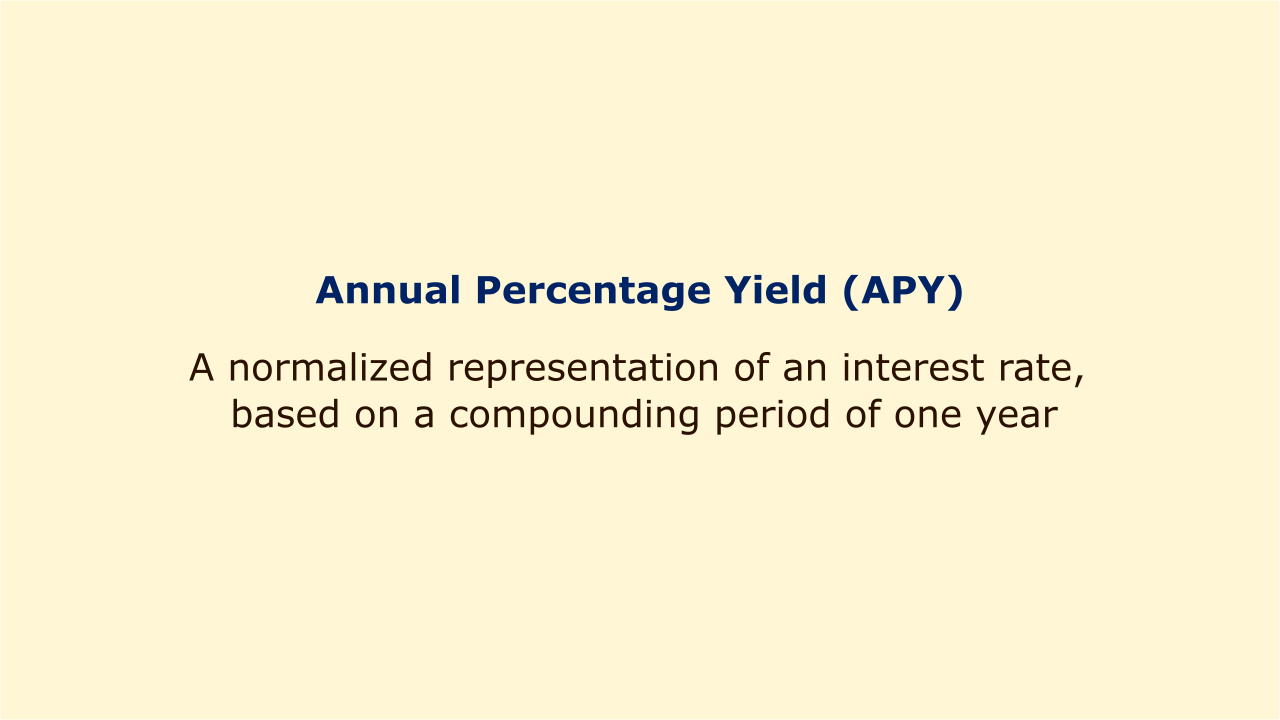# Annual Percentage Yield (APY)Image: Moneybestpal.com

### Annual Percentage Yield (APY) is a normalized representation of an interest rate, based on a compounding period of one year. APY values enable a fair, one-point comparison of various products with various compounding schedules. It does not, however, take into consideration the risk that account fees could reduce the net gain.

Generally speaking, APY refers to the rate that a financial institution pays a depositor, whereas a similar annual percentage rate (APR) refers to the rate that a borrower pays to a financial institution. Banks and other businesses frequently utilize the APY to advertise financial goods without debt (as opposed to the APR because the APY represents the customer receiving a higher return at the end of the term). For instance, a certificate of deposit with a compounded monthly annual percentage rate (APR) of 4.65% would be listed as having a 4.75% APY.

One common mathematical definition of APY uses this effective interest rate formula, but the precise usage may depend on local laws.

APY = (1 + i_nom / N)^N - 1

where i_nom is the nominal interest rate and N is the number of compounding periods per year. For large N we have

APY ≈ e^i_nom - 1

where e is the base of natural logarithms (the formula follows the definition of e as a limit). This is a reasonable approximation if the compounding is daily. Also, it is worth noting that a nominal interest rate and its corresponding APY are very nearly equal when they are small. For example (fixing some large N), a nominal interest rate of 100% would have an APY of approximately 171%, whereas 5% corresponds to 5.12%, and 1% corresponds to 1.005%.

The FDIC Truth in Savings Act of 1991 governs how financial institutions in the United States calculate the APY and the associated annual percentage yield earned.

Different deposit or investment products, such as savings accounts, certificates of deposit, money market accounts, bonds, etc., can be compared using the APY. The depositor will receive more interest in a year the higher the APY. However, other elements like fees, taxes, inflation, liquidity, risk, etc. may also have an impact on an investment's profitability. Investors should therefore take into account all of these factors before selecting a product based just on its APY.
Tags